CBSE Class 10 Sample Paper for 2021 Boards - Maths Standard

Class 10
Solutions of Sample Papers for Class 10 Boards

## The diagrams show the plans for a sun room. It will be built onto the wall of a house. The four walls of the sunroom are square clear glass panels. The roof is made using

• Four clear glass panels, trapezium in shape, all the same size
• One tinted glass panel, half a regular octagon in shape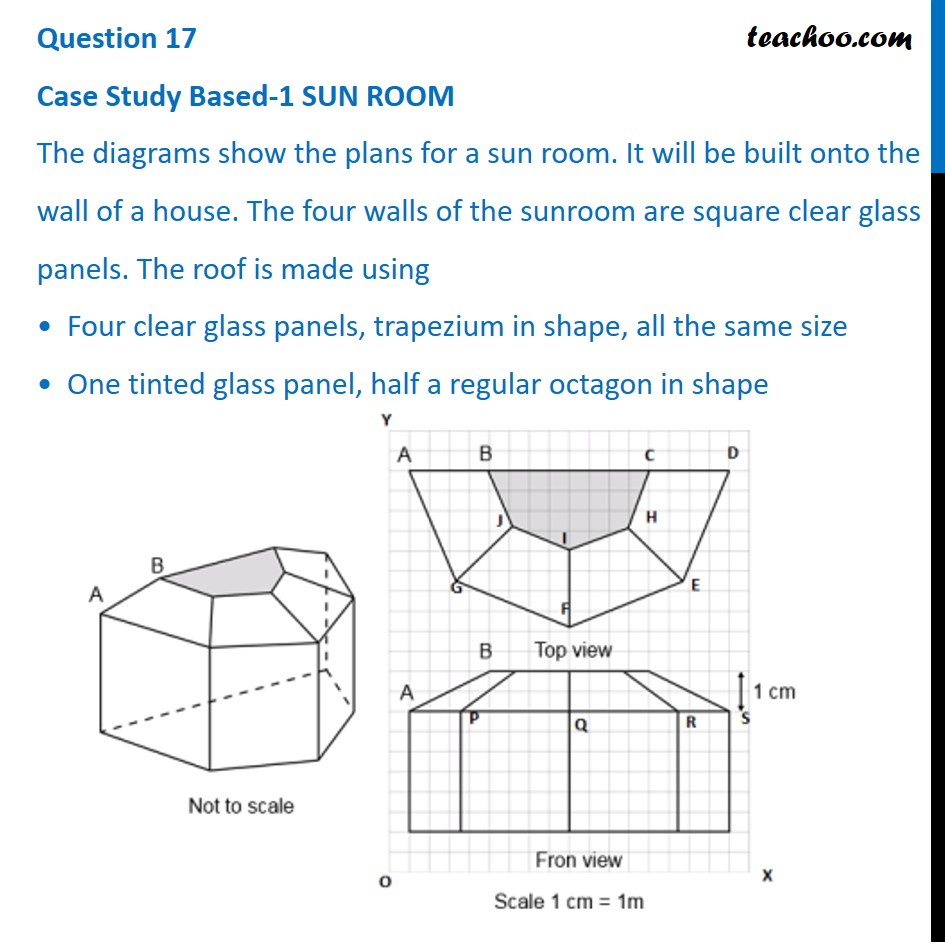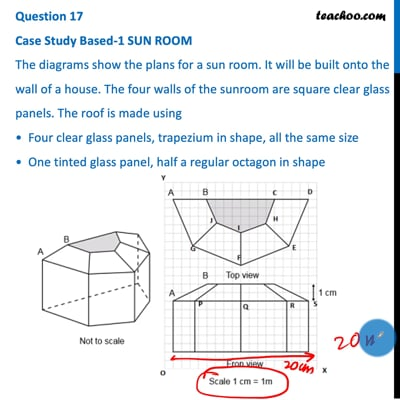This video is only available for Teachoo black users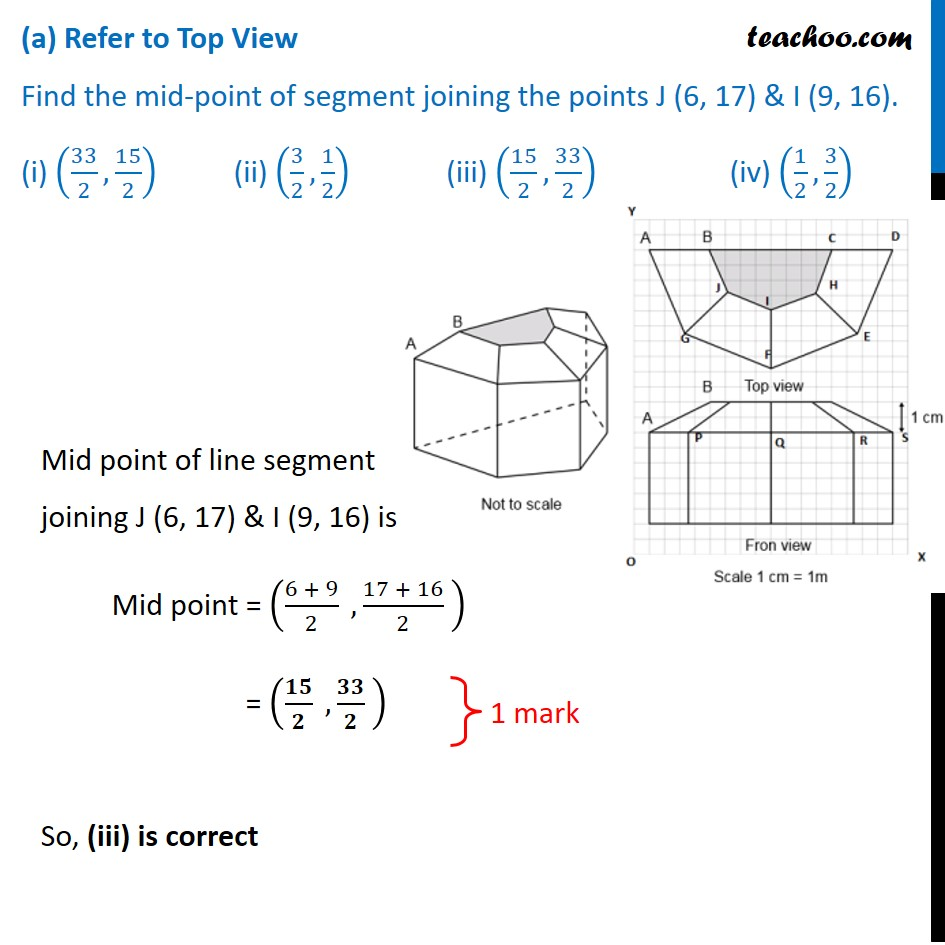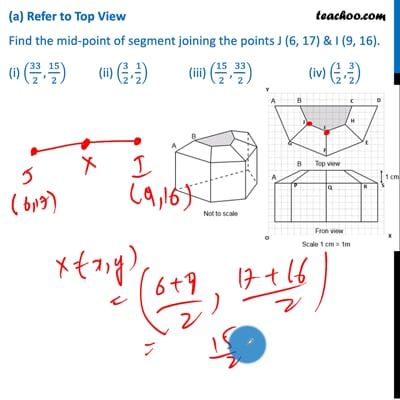This video is only available for Teachoo black users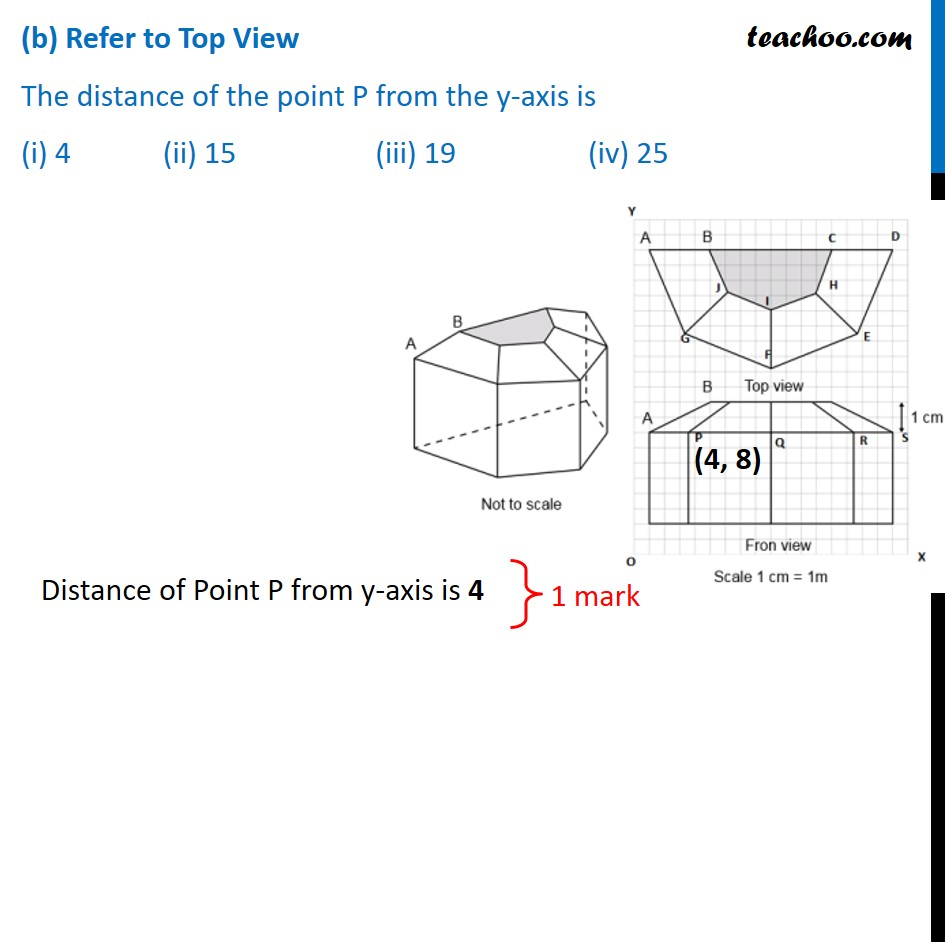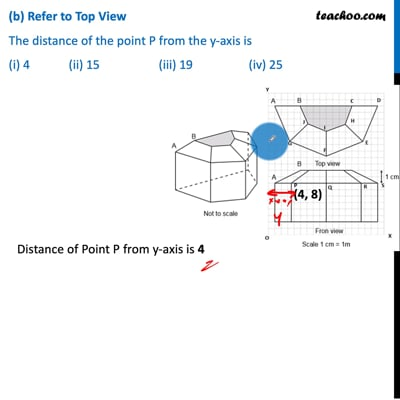This video is only available for Teachoo black users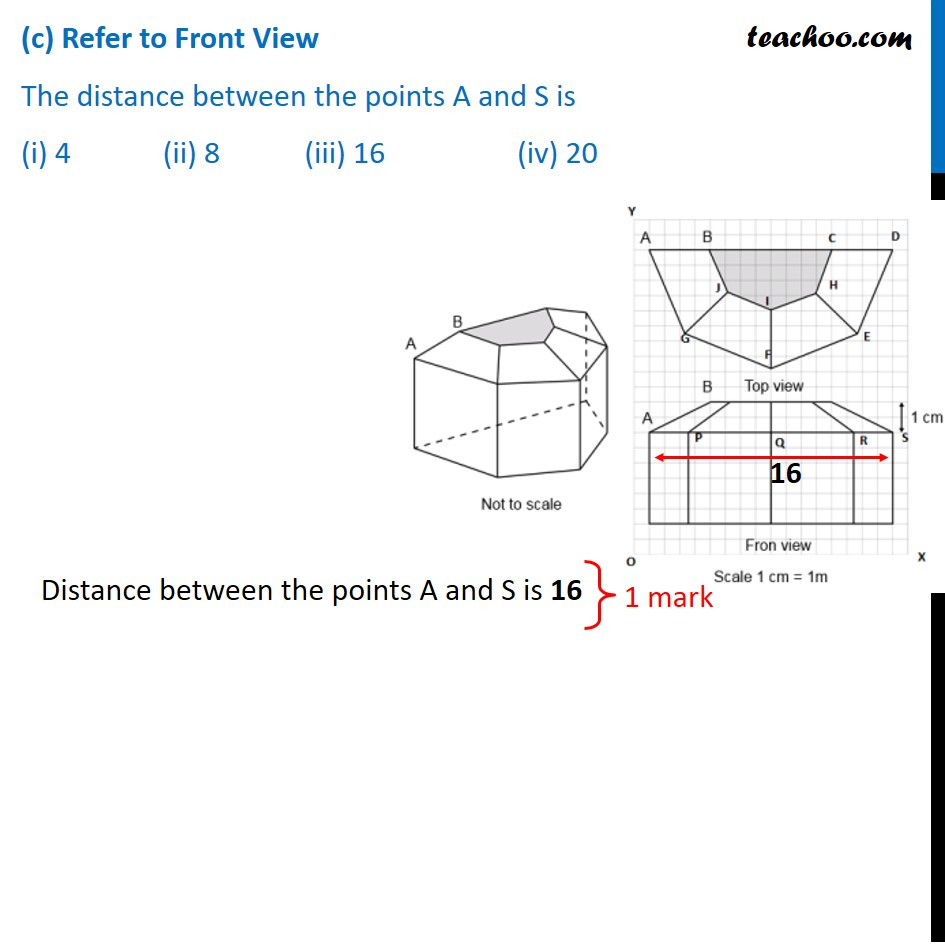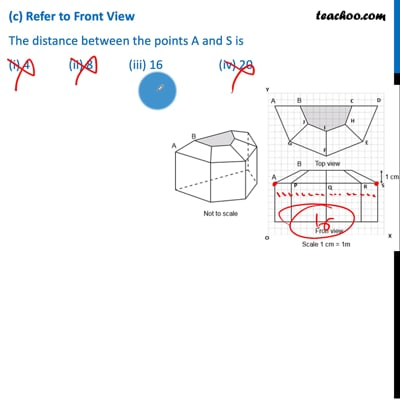This video is only available for Teachoo black users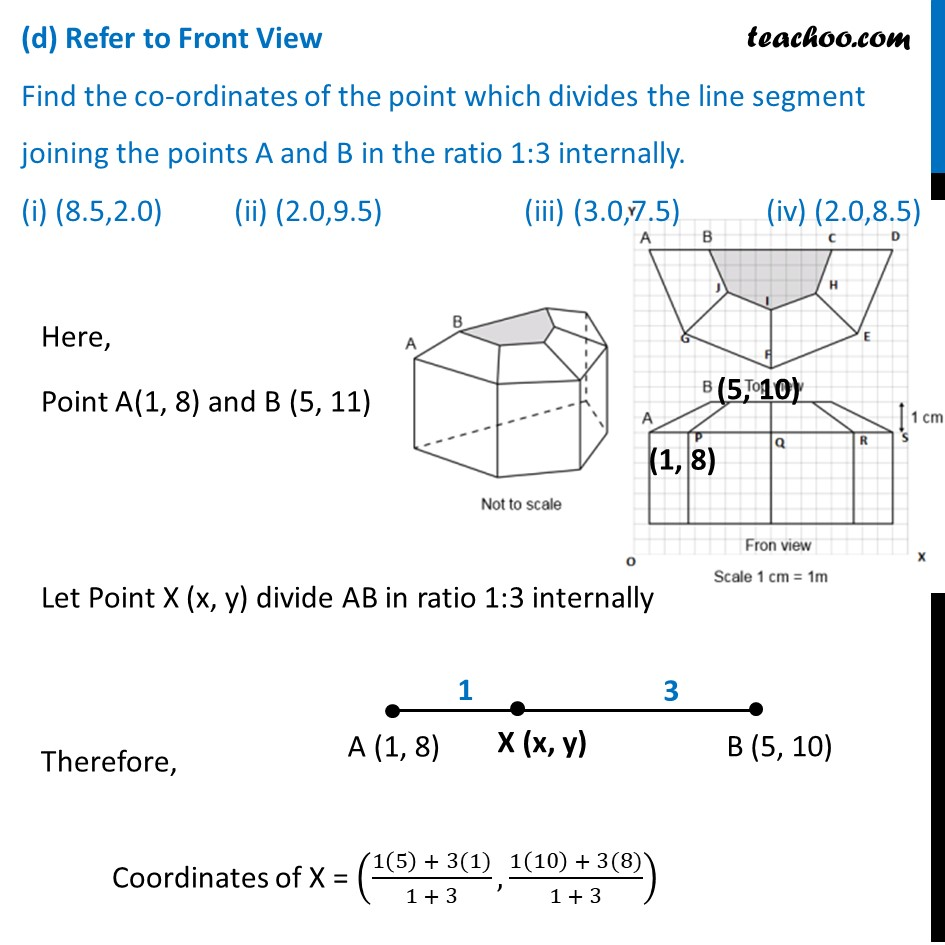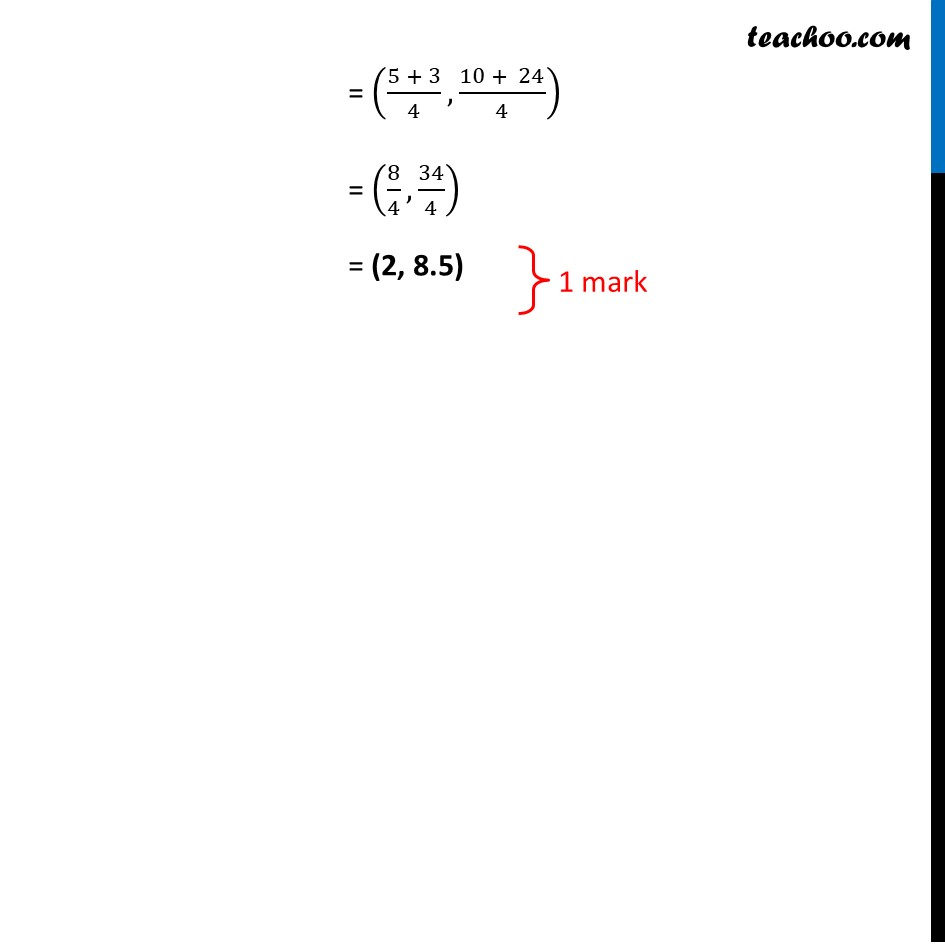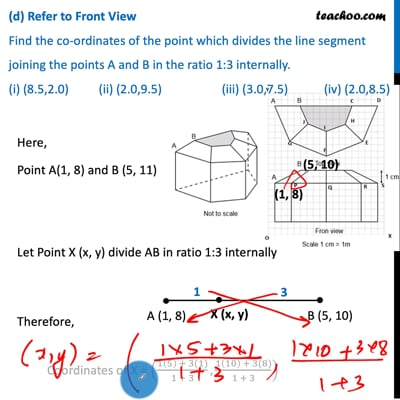This video is only available for Teachoo black users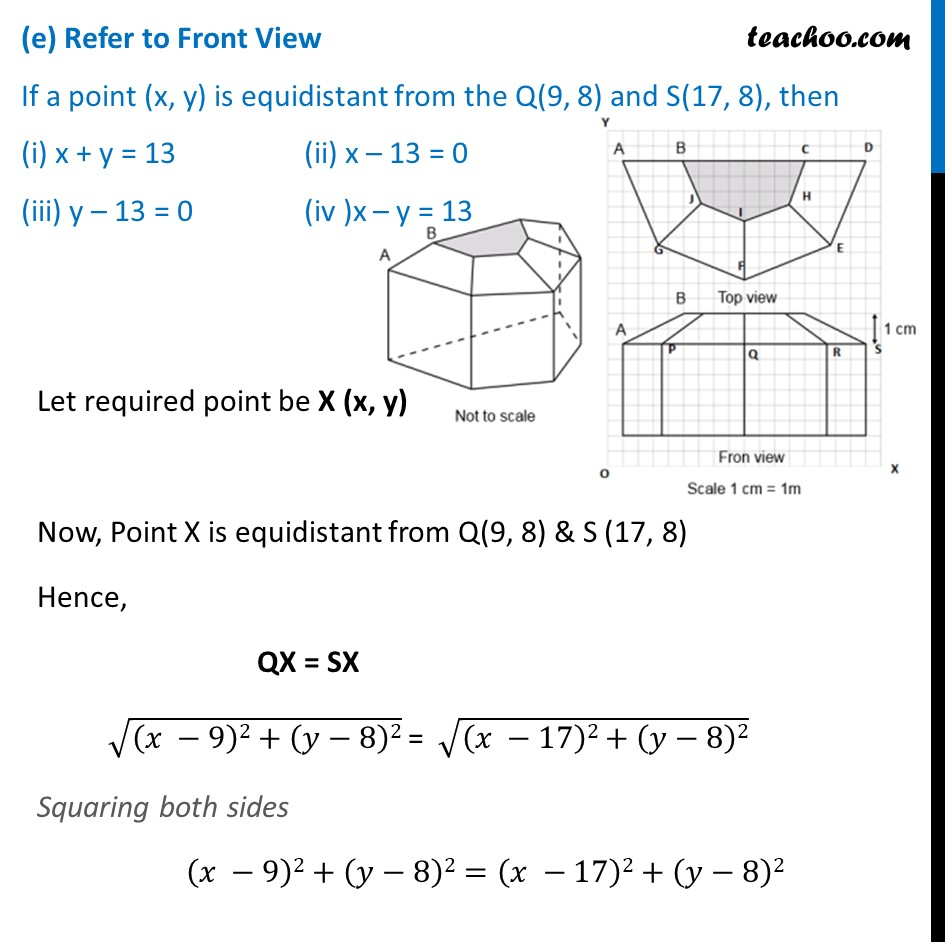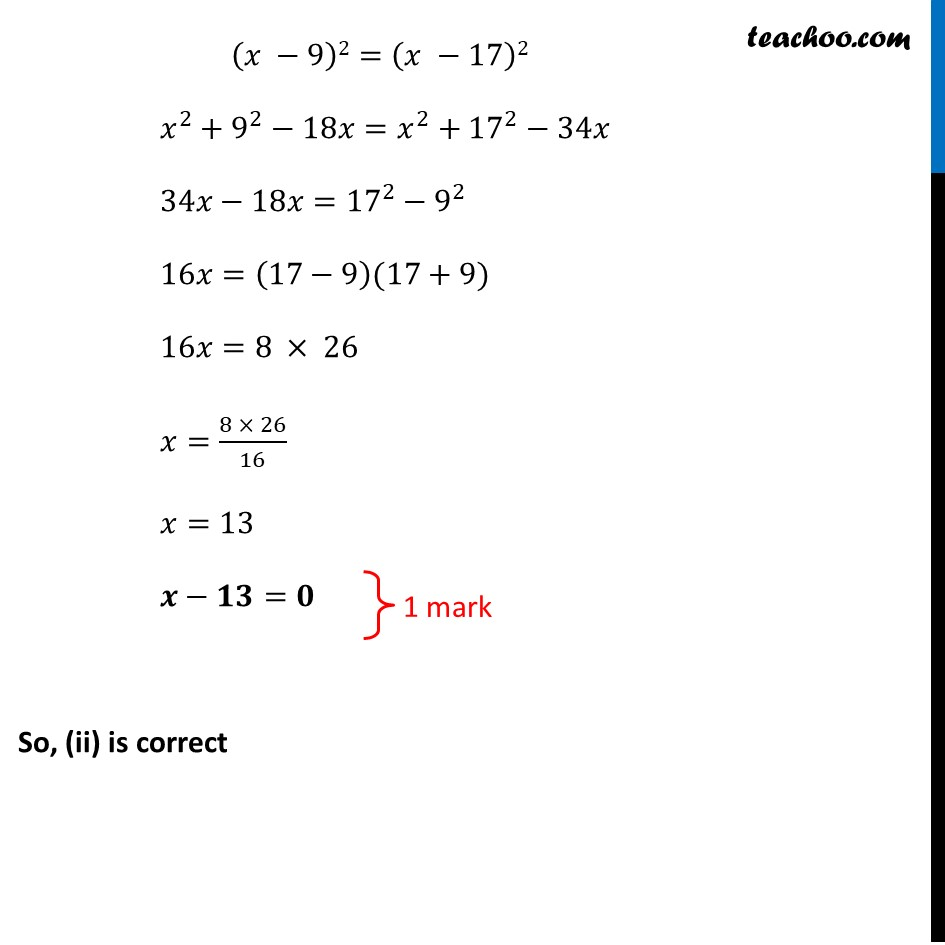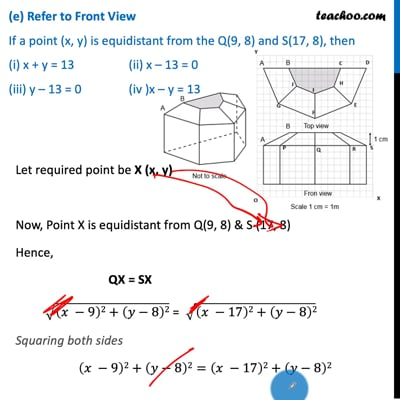This video is only available for Teachoo black users

Note: Check more Case Based Questions for - Coordinate Geometry (Chapter 7 Class 10)

Introducing your new favourite teacher - Teachoo Black, at only ₹83 per month

### Transcript

Question 17 Case Study Based-1 SUN ROOM The diagrams show the plans for a sun room. It will be built onto the wall of a house. The four walls of the sunroom are square clear glass panels. The roof is made using • Four clear glass panels, trapezium in shape, all the same size • One tinted glass panel, half a regular octagon in shape(a) Refer to Top View Find the mid-point of segment joining the points J (6, 17) & I (9, 16). (i) (33/2,15/2) (ii) (3/2,1/2) (iii) (15/2,33/2) (iv) (1/2,3/2) Mid point of line segment joining J (6, 17) & I (9, 16) is Mid point = ((6 + 9)/2 ,(17 + 16)/2 ) = (𝟏𝟓/𝟐 ,𝟑𝟑/𝟐 ) So, (iii) is correct (b) Refer to Top View The distance of the point P from the y-axis is (i) 4 (ii) 15 (iii) 19 (iv) 25 Distance of Point P from y-axis is 4 (c) Refer to Front View The distance between the points A and S is (i) 4 (ii) 8 (iii) 16 (iv) 20 Distance between the points A and S is 16 (d) Refer to Front View Find the co-ordinates of the point which divides the line segment joining the points A and B in the ratio 1:3 internally. (i) (8.5,2.0) (ii) (2.0,9.5) (iii) (3.0,7.5) (iv) (2.0,8.5)Here, Here, Point A(1, 8) and B (5, 11) Let Point X (x, y) divide AB in ratio 1:3 internally Therefore, Coordinates of X = ((1(5) + 3(1))/(1 + 3),(1(10) + 3(8))/(1 + 3)) = ((5 + 3)/4,(10 + 24)/4) = (8/4,34/4) = (2, 8.5) (e) Refer to Front View If a point (x, y) is equidistant from the Q(9, 8) and S(17, 8), then (i) x + y = 13 (ii) x – 13 = 0 (iii) y – 13 = 0 (iv )x – y = 13 Let required point be X (x, y) Now, Point X is equidistant from Q(9, 8) & S (17, 8) Hence, QX = SX √((𝑥 −9)2+(𝑦−8)2) = √((𝑥 −17)2+(𝑦−8)2) Squaring both sides (𝑥 −9)2+(𝑦−8)2=(𝑥 −17)2+(𝑦−8)2 (𝑥 −9)2=(𝑥 −17)2 𝑥^2+9^2−18𝑥=𝑥^2+17^2−34𝑥 34𝑥−18𝑥=17^2−9^2 16𝑥=(17−9)(17+9) 16𝑥=8 × 26 𝑥=(8 × 26)/16 𝑥=13 𝒙−𝟏𝟑=𝟎 So, (ii) is correct